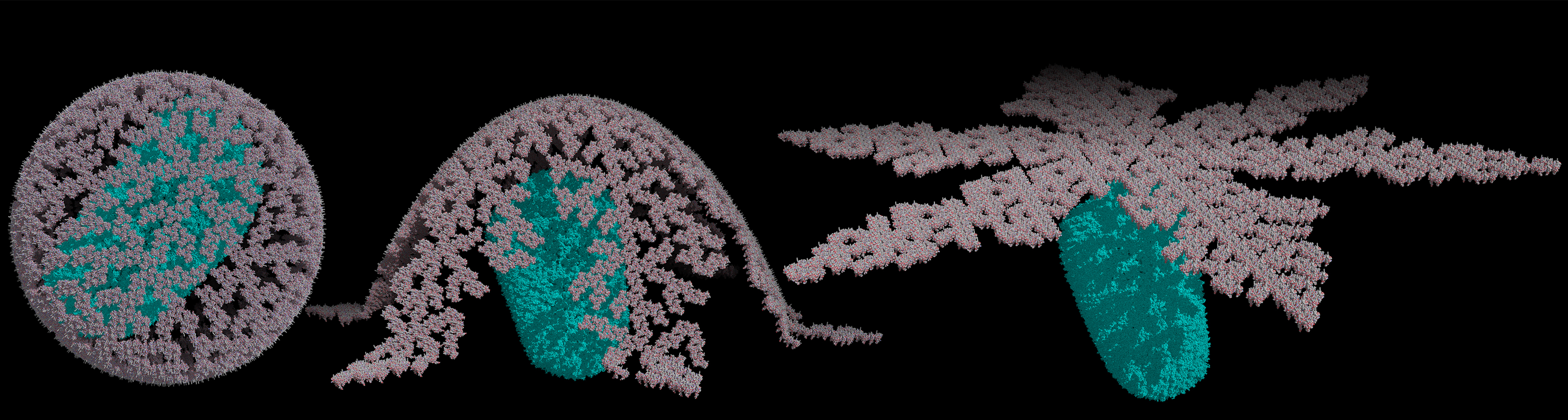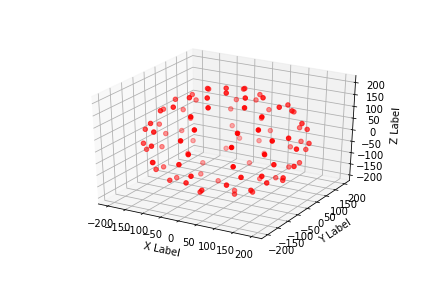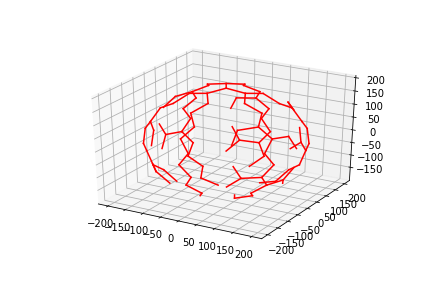# HIV-1 Matrix Builder

## Welcome to our siteThis site will provide you with the tools and step-by-step guide to build HIV-1 Matrix (MA) shells using methods described by Sun W, et al. (2019) Mathematical determination of the HIV-1 matrix shell structure and its impact on the biology of HIV-1. PLOS ONE 14(11): e0224965.

## Technical Overview

We will introduce you to 4 scripts, each of which will allow you to develop unique outcomes:

• Calculate trimer coordinations. Here the position of the center-of-mass for each trimer is placed on a sphere.

• Generate PyMol Model using the output file from coordinates above.

• Generate PyMol Flatten Model using coordinates above.

• Generate tabulated relationship between trimer numbers per viral particle and radius of HIV-1 MA shell.

## System Requirements

### Python 3 support (Recommended)

1. Python 3.5 or greater

2. Anaconda3 > Jupytor NoteBook

3. Python Env Library in Jupytor NoteBook:

4. Basemap 1.2.0 or greater

5. PyMol 2.3.2 or greater

6. Pandas 0.24.2 or greater

## Getting Started

Open Anaconda and create a python 3.5 environment

Install basemap, PyMol, pandas libraries

If a library(s) is not in your Anaconda package, follow the commands bellow``````conda install -c anaconda basemap
conda install -c anaconda pandas
conda install -c schrodinger pymol``````

Then open the main_flow.ipynb file.

You should run the code successfully as shown here.

### Python 2.7 Support

Note: We recommend using the Anaconda environment system described under "Getting Started". The instructions under "Python 2.7 Support" do not need to be followed if you used the Anaconda environment described above.

1. Python 2.7

2. Python Libraries: importlib, matplotlib, mpl_toolkits

3. PyMol 1.72 or greater

• All units are in Angstroms. The interface for the trimer coordination step should look as shown below:
``````>python calculate_trimer_coordinates.py
Input the R:
632
Do you want to use default r = 52.0769942809? (y or n):
y``````

Then you will receive the following message and two output files >> 'points' and 'lines'

``There are 1024 points.``

There are 1024 points' coordinations in the points file and there is another file lines that provides the relationship between the center-of-mass of each trimer and their coordination.

You will be given an option to quickly view what the plots look like. You could `input 3` to quit this script.

``````1 - show and save the lines plot
2 - show and save the points model
3 - quit``````#### Generation of PyMol Model

• Run the following command:
``python pymol_plot_trimer.py``

If your machine supports PyMol, then PyMol will open and run the script within pymol itself.

After the models are generated in PyMol, you may store your models in pdb or pse file formats from PyMol.

#### Generation of Flatten PyMol Model

• Run the following command:
``> python calculate_flatten_trimmer_coordinations.py``

Which will transfer points output file to points-2D

Then you run

``> python pymol_2D_plot_trimer.py``

After the models are generated in PyMol, you may store your models in pdb or pse file formats from PyMol.

#### Relationship Between HIV-1 MA Radius and Number of Trimer Units

• Run the following command:
``> python tabulate_relationship_of_R_and_trimer_number.py``

Then you need to input minimum (Min) Radius, maximum (Max) Radius, and size of the interval steps (interval). The default r referse to the distance between adjacent trimers. All units are in Angstroms.

E.g.:

``````Input the Min Radius: 600
Input the interval: 10
Do you want to use default r = 52.0769942809? (y or n): y``````

The script will output how many trimer units can fit in spheres for each HIV-1 MA radius.

E.g.:

``````909
955
971
1019
1033
1074
1124
1157
1178
1185
1243``````